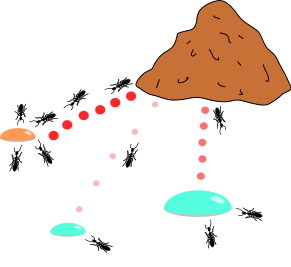# Extended Ant Colony Optimization (gaco)

class pagmo::gaco

Extended Ant Colony Opitmization.Ant colony optimization is a class of optimization algorithms modeled on the actions of an ant colony. Artificial ‘ants’ (e.g. simulation agents) locate optimal solutions by moving through a parameter space representing all possible solutions. Real ants lay down pheromones directing each other to resources while exploring their environment. The simulated ‘ants’ similarly record their positions and the quality of their solutions, so that in later simulation iterations more ants locate better solutions.

In pagmo we propose a version of this algorithm called extended ACO and originally described by Schlueter et al. Extended ACO generates future generations of ants by using the a multi-kernel gaussian distribution based on three parameters (i.e., pheromone values) which are computed depending on the quality of each previous solution. The solutions are ranked through an oracle penalty method.

This algorithm can be applied to box-bounded single-objective, constrained and unconstrained optimization, with both continuous and integer variables.

M. Schlueter, et al. (2009). Extended ant colony optimization for non-convex mixed integer non-linear programming. Computers & Operations Research.

Note

The ACO version implemented in PaGMO is an extension of Schlueter’s originally proposed extended ACO algorithm. The main difference between the implemented version and the original one lies in how two of the three pheromone values are computed (in particular, the weights and the standard deviations).

Public Types

typedef std::tuple<unsigned, vector_double::size_type, double, unsigned, double, double, double> log_line_type

Single entry of the log (gen, m_fevals, best_fit, m_ker, m_oracle, dx, dp)

typedef std::vector<log_line_type> log_type

The log.

Public Functions

gaco(unsigned gen = 1u, unsigned ker = 63u, double q = 1.0, double oracle = 0., double acc = 0.01, unsigned threshold = 1u, unsigned n_gen_mark = 7u, unsigned impstop = 100000u, unsigned evalstop = 100000u, double focus = 0., bool memory = false, unsigned seed = pagmo::random_device::next())

Constructs the ACO user defined algorithm for single-objective optimization.

Parameters
• gen[in] Generations: number of generations to evolve.

• ker[in] Kernel: number of solutions stored in the solution archive.

• q[in] Convergence speed parameter: this parameter is useful for managing the convergence speed towards the found minima (the smaller the faster).

• oracle[in] Oracle parameter: this is the oracle parameter used in the penalty method.

• acc[in] Accuracy parameter: for maintaining a minimum penalty function’s values distances.

• threshold[in] Threshold parameter: when the generations reach the threshold then q is set to 0.01 automatically.

• n_gen_mark[in] Standard deviations convergence speed parameter: this parameters determines the convergence speed of the standard deviations values.

• impstop[in] Improvement stopping criterion: if a positive integer is assigned here, the algorithm will count the runs without improvements, if this number will exceed impstop value, the algorithm will be stopped.

• evalstop[in] Evaluation stopping criterion: same as previous one, but with function evaluations.

• focus[in] Focus parameter: this parameter makes the search for the optimum greedier and more focused on local improvements (the higher the greedier). If the value is very high, the search is more focused around the current best solutions.

• memory[in] Memory parameter: if true, memory is activated in the algorithm for multiple calls

• seed – seed used by the internal random number generator (default is random).

Throws

std::invalid_argument – if acc is not $$>=0$$, impstop is not a positive integer, evalstop is not a positive integer, focus is not $$>=0$$, ker is not $$>=2$$, oracle is not positive, threshold is not $$\in [1,gen]$$ when $$memory=false$$ and $$gen!=0$$, threshold is not $$>=1$$ when $$memory=true$$ and $$gen!=0$$, q is not $$>=0$$

population evolve(population) const

Algorithm evolve method.

Evolves the population for the requested number of generations.

Parameters

pop – population to be evolved

Throws
• std::invalid_argument – if pop.get_problem() is multi-objective or stochastic

• std::invalid_argument – if the population size is not at least 2

• std::invalid_argument – if kernel parameter is bigger than the population size

Returns

evolved population

void set_seed(unsigned)

Sets the seed.

Parameters

seed – the seed controlling the algorithm stochastic behaviour

inline unsigned get_seed() const

Gets the seed.

Returns

the seed controlling the algorithm stochastic behaviour

inline void set_verbosity(unsigned level)

Sets the algorithm verbosity.

Sets the verbosity level of the screen output and of the log returned by get_log(). level can be:

• 0: no verbosity

• >0: will print and log one line each level generations.

Example (verbosity 1):

*Gen:        Fevals:          Best:        Kernel:        Oracle:            dx:            dp:
1              0        179.464             13            100        4.33793          47876
2             15         14.205             13            100        5.20084        5928.12
3             30         14.205             13         14.205        1.24173        1037.44
4             45         14.205             13         14.205        3.05807         395.89
5             60        7.91087             13         14.205       0.711446        286.599
6             75        2.81437             13        7.91087        5.80451        71.8174
7             90        2.81437             13        2.81437        1.90561        48.3829
8            105        2.81437             13        2.81437         1.3072        26.9496
9            120         1.4161             13        2.81437        1.61732        10.6527
10            150         1.4161             13         1.4161        2.54262        3.67034

Gen, is the generation number, Fevals the number of function evaluation used, Best is the best fitness function value found until that generation, Kernel is the kernel size, Oracle is the oracle parameter value, dx is the flatness in the individuals, dp is the flatness in the penalty function values.

Parameters

level – verbosity level

inline unsigned get_verbosity() const

Gets the verbosity level.

Returns

the verbosity level

inline unsigned get_gen() const

Gets the generations.

Returns

the number of generations to evolve for

void set_bfe(const bfe &b)

Sets the batch function evaluation scheme.

Parameters

b – batch function evaluation object

inline std::string get_name() const

Algorithm name.

Returns the name of the algorithm.

Returns

 std::string  containing the algorithm name

std::string get_extra_info() const

Extra info.

Returns extra information on the algorithm.

Returns

an  std::string  containing extra info on the algorithm

inline const log_type &get_log() const

Get log.

A log containing relevant quantities monitoring the last call to evolve. Each element of the returned std::vector is a gaco::log_line_type containing: gen, m_fevals, best_fit, m_ker, m_oracle, dx, dp as described in gaco::set_verbosity

Returns

an std::vector of gaco::log_line_type containing the logged values gen, m_fevals, best_fit, m_ker, m_oracle, dx, dp